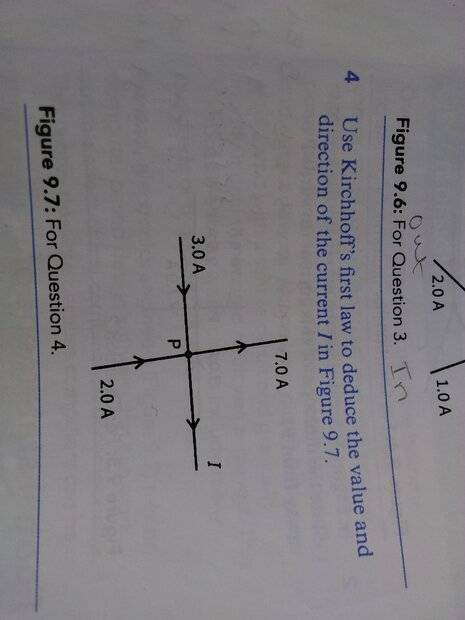# Using Kirchhoff's law, deduce the value and direction of the current

• haha0p1
In summary, according to Kirchhoff's law, the value and direction of current I can be deduced as -2A entering from the top of the diagram. This may seem counterintuitive, but it is due to the nature of Kirchhoff's law and the fact that the positive or negative designation of current is based on the direction of the arrow on the diagram, not the actual direction of the current itself. Therefore, in this case, the current is actually entering the wire from the top, even though the arrow is pointing in the opposite direction. This satisfies Kirchhoff's law, as the current entering and exiting the wire is balanced.

#### haha0p1

Homework Statement
Use Kirchhoff's law to deduce the value and direction of the current I.
Relevant Equations
Current entering a point is equal to current exiting a point
3 A+ 2 A = 7 A+ I
I = -2A
How the current can be in negative direction? If the sign if negative, doesn't it mean that the current will move towards the point P? Also If I am taking the 2A to be positive then Kirchhoff's law isn't satisfied as 5A≠7A#### Attachments

haha0p1 said:
Homework Statement:: Use Kirchhoff's law to deduce the value and direction of the current I.
Relevant Equations:: Current entering a point is equal to current exiting a point

3 A+ 2 A = 7 A+ I
I = -2A
How the current can be in negative direction? If the sign if negative, doesn't it mean that the current will move towards the point P?
The arrow on the diagram indicates the positive direction for current ##I##. It's doesn't imply the current itself is in the positive direction.

••PhDeezNutz and haha0p1
PeroK said:
The arrow on the diagram indicates the positive direction for current ##I##. It's doesn't imply the current itself is in the positive direction
Even If I take the value of I to be 2 A, Still my current entering the wire and current exiting the wire is not the same as 3+5=7+2
And 5≠9

haha0p1 said:
Even If I take the value of I to be 2 A
Why would you do that?

PeroK said:
Why would you do that?
Because The question is asking to find value of I which is coming 2

haha0p1 said:
Because The question is asking to find value of I which is coming 2
##I \ne 2## as you yourself have already established. And, you seem to know that ##I = -2## but have decided that current must be positive. That puts you in an impossible position in this case.

•SammyS
think of it this way:

If you designate a current leaving a node as positive and it mathematically turns out negative via KCL then that current is actually entering.

What’s really happening is that

3A is entering from the right
2A is entering from the bottom
2A is entering from the left

7A is leaving from the top

•haha0p1 and PeroK